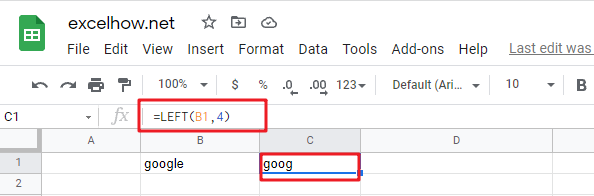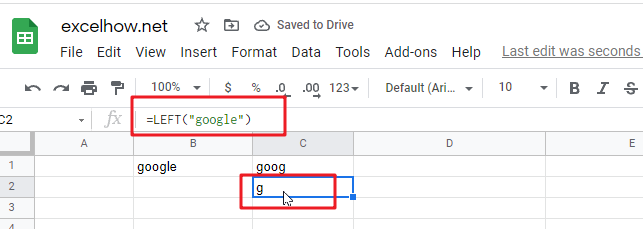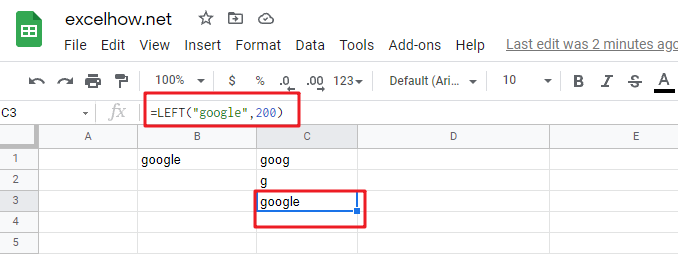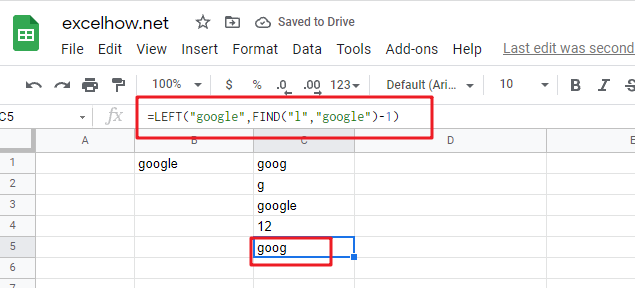# ExcelHow

This post will guide you how to use Google Sheets LEFT function with syntax and examples.

## Description

The Google Sheets LEFT function returns a substring (a specified number of the characters) from a text string, starting from the leftmost character.

The LEFT function can be used to extract a given number of characters from the leftmost of a text string in google sheets. It returns a substring from the beginning of a specified string.

The LEFT function is a build-in function in Google Sheets and it is categorized as a Text Function.

## Syntax

The syntax of the LEFT function is as below:

= LEFT(text,[num_chars])

Where the LEFT function arguments are:

• Text -This is a required argument. The text string that you want to extract from.
• num_chars-This is an optional argument. It will specify the number of characters that you want to extract from Text string.

Note:

• The `Num_chars` value must be greater than or equal to zero. If `Num_chars` is greater that the length of text string, it will return all of text string. If `Num_chars` value is omitted, it is set to be 1 by default.
• If `Num_chars` is equal to 0, it will cause LEFT function to extract the empty string.

## Google Sheets LEFT Function Examples

The below examples will show you how to use google sheets LEFT Text function to extract the leftmost substring from a text string.

#1 To extract the leftmost 4 characters in the B1 cell, just using formula:

`=LEFT(B1,4)`#2 If you do not provide num_chars argument, it will use the default value as 1, using the following formula:

`=LEFT(“google”)`#3 If Num_chars exceeds the text string length, the LEFT function will return the entire string:

`=LEFT("google",200)`#4 If Text value is a numeric value, and the LEFT function will return a text value:

`=LEFT(1234,2)`#5 You can also use the LEFT function in combination with the FIND function to create a complex formula to extract a specific text string. The FIND function will get the position of the given charcter in text string, and the LEFT function returns all text characters to the left of that position in google sheets.

`=LEFT("google",FIND("l","google")-1)`Note:

You can use the LEFT function to extract substring from the left side of a text string in Google Sheets. And you can also use another function called RIGHT function to extract a substring starting from the right side of the text string. And if you wish to extract a substring starting from the middle position of text string, you can use the MID function.